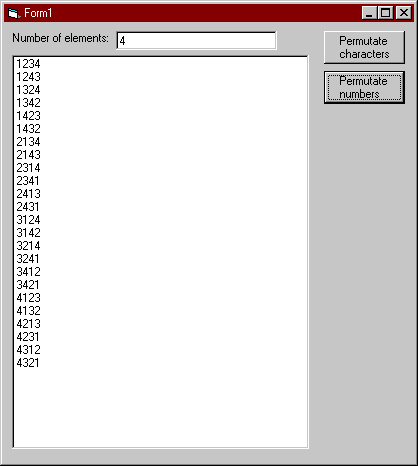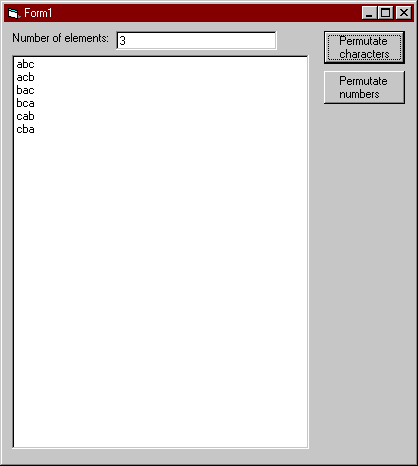Permutating an array
This article will demonstrate the technique used to permutate an array, and how to apply this technique in a real-life example. It assumes familiarity with common programming concepts as arrays and recursion.
Related items
Author: Jens G. Balchen

## Introduction

A permutation, by dictionary standards, is an ordered arrangement of a set of objects, and permutating is the act or process of changing the lineal order of an ordered set of objects. In other words, it's re-ordering the elements of a list, like an array. So how is this valuable?

In a recent project, I had to write a procedure to estimate the execution time of a series of component calls. Based on statistics gathered from previous executions, I could approximate the time spent in each call. The real issue was, which order of execution is most optimal? The output and execution time of each component being predictable, all I had to do was determine all possible orders of execution, and then calculate the execution time. Sound familiar?

Determining all possible orders of execution was as simple as putting the component ids into an array and permutating it. The actual array was an array of Long, which is what I'll be using in this article.

## The Theory

Imagine an array, consisting of the elements a, b, and c. We'll write this as (a,b,c). When we permutate this array, we want to re-arrange the elements, so that the a is in a different position relative to b and c. Of course, when we're done, we want to end up with the following:

(a,b,c)
(a,c,b)
(b,a,c)
(b,c,a)
(c,a,b)
(c,b,a)

If you look carefully, you'll see a pattern here: The first element is a,a,b,b,c,c. It seems like each element will appear as the first element the same number of time. This is logical -- we don't want the a to appear more than its share. However, it also seems like each element will appear as many times as there are unique combinations of the other elements. So the a appears two times because there are two combinations of (b,c). This can be tested by extending the array to four elements; (a,b,c,d). I'll leave that as an exercise.

So, if you want to create all permutations of the array (a,b,c), you take each element and place it as the first element of a series of new arrays, in numbers being equal to the number of permutations of the remaining elements. With the a as the first elements, this yields

(a,x,x)
(a,x,x)

Then you have fill in the last two spots. What you have now is a slightly shorter array. In the case of a being the first element, the array has been reduced to (b,c). To permutate (b,c), you take each element and place it in the middle of the arrays starting with a.

(a,b,x)

Now you're left with an even shorter array: (c). This one's very easy. All possible combinations of the array (c) is: (c). So with only one combination left, this one has to go in the final position.

(a,b,c)
(a,x,x)

OK, first permutation is done. Actually, this is what we started out with, but you've got to admit that it is a valid combination. Let's backtrack to where we appointed b as the middle element. We were taking each element of the array (b,c) and placing it in the middle. We already did b, which means c is up next.

(a,c,x)

Aha. The remainder is (b). (b) is no more special than (c), and goes in the final position as the only possible combination of itself. Once again, we have found a permutation.

(a,b,c)
(a,c,b)

So, two permutations found, both starting with a. Remember that we're doing one element at a time, always trying to permutate the elements that are left in the array. This time, b is the first element, and is taken out so that (a,c) remain. Permutating (a,c) with the same method as with (b,c) will yield

(b,a,c)
(b,c,a)

No surprises there. If you try to visualize how this actually folds out, it would look like this:

```a
b
c
c
b

b
a
c
c
a

c
a
b
b
a
```

Being familiar with recursion, you will have spotted the recursive nature of the problem by now. By breaking the array (a,b,c) down into (a)(b,c), we have created a new array (b,c) to permutate. Applying the method to (b,c) will break it down further into (b)(c), and these arrays are trival to permutate. In the end, it all breaks down to two things: taking one element at a time, and putting elements in the next position.

## The Implementation

If by now you don't understand the theory, perhaps reading and running the code will help. Recursion is learned best by experience, and stepping through the code in the debugger may be helpful.

There are certain things the procedure must know to permutate an array. Most importantly, it needs the array itself. But in order to put elements in their right position in the re-ordered array, it must be able to figure out what that position is. Finally, it needs a way to store two things: 1) while building one permutation, it must remember the array in which the permutation is stored. 2) it must be able to store a list of permutations, and return this list when it's done.

To calculate the right position of each element, we require the length of the array to permutate. This way, we can calculate the position by doing

```position = (permutated_array_length - current_array_length) + 1
```
If you look in the theory section, you'll see that when the array has its original length (3), the first item goes in position 1 (3 - 3 + 1 = 1). When the array has been decreased to 2, the first item goes in position 2 (3 - 2 + 1 = 2). Finally, the last item goes in position 3 (3 - 1 + 1 = 3).

To store all the possible permutations, I chose a Collection object. The Collection is passed to the procedure by the caller, and is populated with arrays. Since we know when a permutation is done (having filled in the last element), we can add the permutation to the Collection at that time.

The last thing we need is a way of remembering the array that we're currently filling in with permutated elements. Looking back at the theory section, the procedure will call itself three times when the array contains three elements. Between these three calls, the permutation will be partially completed. To remember it, the procedure will need a temporary array to work with. To avoid complexety, we assume this array is also produced as a parameter to the procedure, allowing it to be passed between procedure calls, and that it has the same dimensions as the array we want to permutate.

```
Public Sub Permutate( _
ByVal ArrayCount As Long, _
ByRef Elements() As Long, _
ByRef Order() As Long, _
ByRef Orders As Collection)

End Sub

```
This is the procedure skeleton. ArrayCount is the number of elements in the original array to permutate, Elements() is the array to permutate (remember, this will grow shorter as we work, so the ArrayCount parameter cannot be deduced from the length of Elements). Order is the temporary array where we store one permutation, and Orders is the Collection where we store all permutations found.

The code itself is very simple, with 12 lines making up the main body. The rest is comments and declarations.

```
Public Sub Permutate( _
ByVal ArrayCount As Long, _
ByRef Elements() As Long, _
ByRef Order() As Long, _
ByRef Orders As Collection)

Dim Position   As Long
Dim Element    As Long
Dim i          As Long
Dim ArrayLen   As Long

' The length of the Elements array. We need this
' for our calculations later on.
ArrayLen = (UBound(Elements) - LBound(Elements) + 1)

' Position in the Order array of the first element in
' the permutated arrays.
'
' Example: Given the array(a,b,c,d), where we want to permutate
' (b,c,d), the position in the new array for the first element
' will be 2 (since (a) will take up the first position).
' Likewise, when we permutate (c,d), the position of the first
' element will be 3, since the first two spots are taken by
' (a,b).

Position = ArrayCount - ArrayLen + 1

If ArrayLen = 1 Then
' The most primitive array we will permutate.
' The result is the array itself, and the result
' is inserted in the last position of the Order array.
Order(Position) = Elements(LBound(Elements))

' This Order is now complete, since the final element has
' been filled in.
Else
' The permutation of Elements is each distinct Element
' + all permutations of the remaining elements.
For i = LBound(Elements) To UBound(Elements)
Element = Elements(i)
Order(Position) = Element
Permutate ArrayCount, RemoveFromArray(Elements, Element), Order, Orders
Next i

End If

End Sub
```
The only code we're missing is RemoveFromArray. VB has no built-in function to handle this, so I wrote a simple function to do it.
```
Public Function RemoveFromArray(ByRef Elements() As Long, ByVal Element As Long) As Long()

Dim NewArray() As Long
Dim i          As Long
Dim newi       As Long

' Will create a new array where Element has been left out.

ReDim NewArray(LBound(Elements) To UBound(Elements) - 1)
For i = LBound(Elements) To UBound(Elements)
If Elements(i) <> Element Then
newi = newi + 1
NewArray(newi) = Elements(i)
End If
Next

RemoveFromArray = NewArray

End Function
```
That's all there is to it.

## Testing the code

I wrote a simple test application that will accept a number (of elements) as input, and will output a list of arrays consisting of either numbers or characters. The images below illustrate how the application works.